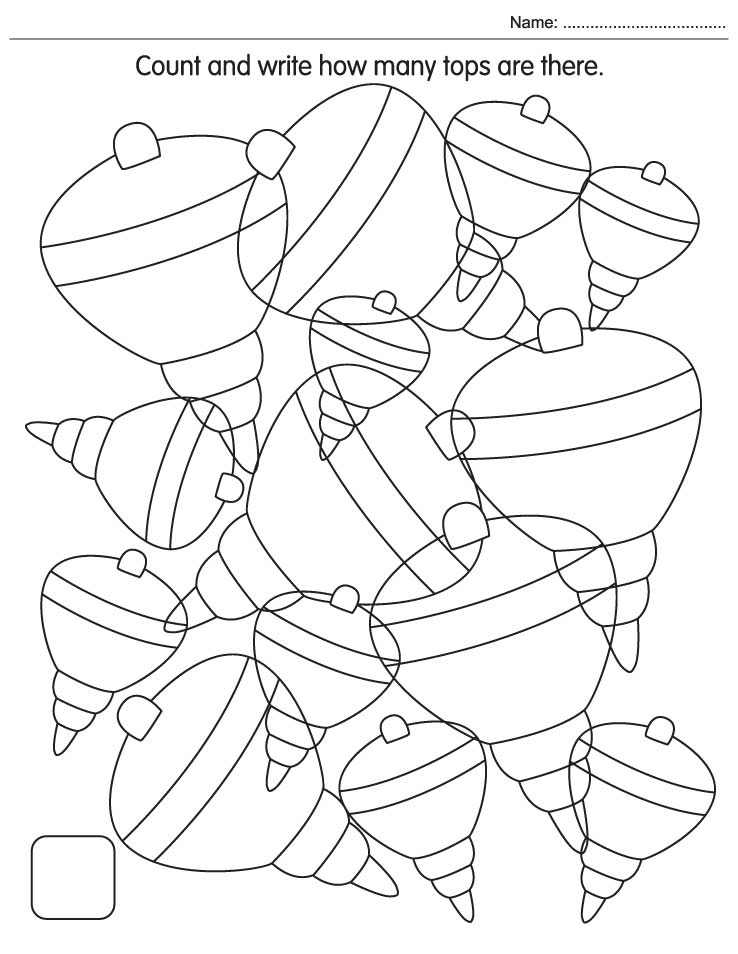# Home

## Worksheet Category

### Count and write

Page of 20Page of 20

### Count and write how many tops are thereSimple & Easy Maths Worksheets 95Count and write how manySolve the number puzzlesNumber the picture pieces in the correct orderCount and write starsLook at the three sets of animals belowWrite the numbersComplete the sums using the codesSolve the sumsCross out the following as directedDraw a lineFollow the table of 8 and join the starsFind the totalColor the pictures that have even numbersJoin the dots from 1-20Mark the numbers which are not in orderEncircle the number 6 and count how many times it appearsColor the given number of objectsDraw, color and complete the sum